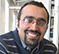# Analytic Signal

In communication theory and modulation theory we always deal with two phases: In-phase (I) and Quadrature-phase (Q). The question that I will discuss in this blog is that why we use two phases and not more.

Any real band-limited signal along with its Hilbert transformed pair form an analytic signal. We normally use the analytic signal for modulation. A modulated signal is actually a carrier or the sine signal that one attribute of it is changing with time which is our signal. These attributes might be amplitude, phase or frequency. A sine signal has not any other parameter to change. Albeit, we can discuss about modulating the phase second, third,... derivative or changing the amplitude derivatives. But current modulations rely on amplitude and phase and phase first derivative (frequency).

The analytic signal in the pass-band is the summation of the pass-band signal and its Hilbert transform. The envelope of this modulated signal will be the real signal.

$$x(t)={ I cos(\omega t) -Q sin(\omega t)}.\tag {1}$$

Now the question is that why in current communication we are limited to this representation?

The answer lies in the definition of Fourier transform. As we know the Euler equation

$$\exp(j \theta) ={cos(\theta) + j sin( \theta)}.\tag {2}$$

since there are only two basis functions that are orthogonal to each other, we can only have two phases in the communications. If we extend the idea to more than two dimensions we will have the Quaternions and the hyper-analytic signals . The reason that why we use just use $sin$ and $cos$ lies in their simplicity and orthogonality. Any other set of orthogonal pairs in two dimensions, based  on the Fourier theorem can be described in terms of these two functions. To extend the idea of analytic signal we have to follow the 4-D Hamilton space or higher dimensional spaces to have more phases in the modulation theory.

There is a question: Why we are limited to circular trigonometry? Does non-circular trigonometry lead to more efficient and faster communications?

One answer to this question is that our electromagnetic theory has been developed for sine waves. And if the theory changes for non-circular waves, these waves may change our communications.

 Sangwine, S.J.; Le Bihan, N., "Hypercomplex analytic signals : Extension of the analytic signal concept to complex signals," in Signal Processing Conference, 2007 15th European , vol., no., pp.621-624, 3-7 Sept. 2007

[ - ]
Comment by April 11, 2017Your definition of Equation (1) is a bit confusing.  You say it's "the complex envelope of this modulated signal is the real signal".  However, from my understanding the complex envelope is complex not real.  Equation (1) uses I and Q, which I think is the baseband complex envelope defined in (2.1-10) and (2.1-11) of Dig. Comm. 5th ed., J. Proakis and Salehi.  Can you help clear this for me?  Thanks.

[ - ]
Comment by April 11, 2017Hello,

The definition of the complex envelope can be found in .

The idea of the envelope of a modulated carrier which is real in analytic signals is extended to the complex envelope of hyper analytic signal which is complex. Please refer to  for more details.

Regards,

Mehdi

[ - ]
Comment by April 11, 2017This is clearer but your statement in the article is then imprecise. Shouldn't it be changed to:  "the envelope of this modulated signal is the real signal"?

[ - ]
Comment by April 11, 2017Thanks for the comment. I will fix that.

Regards,

Mehdi

[ - ]
Comment by November 14, 2022Is there any application of analytic signal in the field of electrical power system ?

To post reply to a comment, click on the 'reply' button attached to each comment. To post a new comment (not a reply to a comment) check out the 'Write a Comment' tab at the top of the comments.